# Venn Diagrams: Subset, Disjoin

#### Video Lesson on Venn Diagrams: Subset, Disjoin

This lesson video Not available at this time available video coming soon

### Venn Diagrams: Subset, Disjoint, Overlap, Intersection & Union

The Venn diagram was introduced by John Venn. Yes, the Venn diagram is named after a real person! His idea was to show sets in terms of pictures. The Venn diagram is now used in many fields, including mathematics. Let's take a look at John Venn's idea.

### Set Review

Before we get into Venn diagrams, let's review sets. A set is a collection of elements. An element is a collection of anything - numbers, letters, words, objects. Using math symbols, the symbol below means element.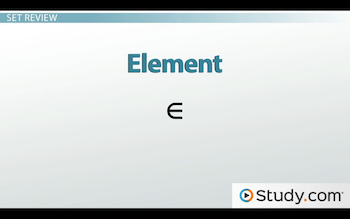Mathematical symbol for element

A subset is a special, funny set. If every element in one set is included in another set, they are called subsets. Using math symbols, the symbol below means subset.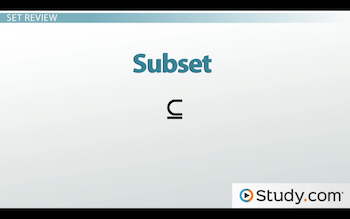Mathematical symbol for subset

Let's say our universe is the things, or elements, in my apartment. So, our universal set U = {fork, spoon, knife, spatula, whisk, plate, cup, napkin, tablecloth, television, couch}.

Let set K be a list of utensils in my kitchen. K = {fork, spoon, knife, spatula, whisk}.

Let set T be a list of things, or elements, on my table. T= {plate, fork, knife, cup, napkin, tablecloth}.

So, we can say that a fork is an element of K. Using math symbols, we would write: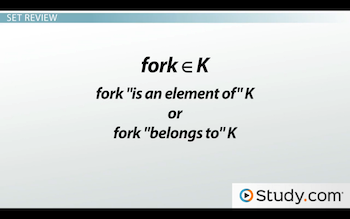Fork is an element of K, using math symbols.

This is read, 'Fork is an element of K,' or, 'Fork belongs to K.' That makes sense because the fork belongs in the kitchen.

### Set Operations

Let's use sets K and T to review set operations.

### Union

Union is when two or more sets are united, or added together.

K union T = all of the elements of set K or all of the elements in set T, and that would equal {fork, spoon, knife, spatula, whisk, plate, cup, napkin, tablecloth}.

The 'or' tells us that it is any object in set K, the kitchen, or set T, on the table.

### Intersection

Intersection is when two or more sets intersect, or are common objects, in sets.

K intersects T = all of the numbers in set K and set T, and that equals {fork, knife}.

The 'and' tells us that it is in the kitchen and on the table at the same time.

### Complement

Complement is when a set is subtracted or not included in the set.

K' = everything not in the kitchen, not in set K, and that equals {plate, cup, napkin, tablecloth, television, couch}.

### Venn Diagram

A Venn diagram can show us the sets and operations nicely in picture form. Let's look at union, intersection and complement using a Venn diagram. We're going to continue using set K and set T. Below is what a Venn diagram showing the sets K and T looks like: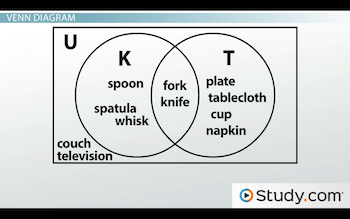Venn diagram of sets K and T

Let's examine how the Venn diagram is created.

There's a circle for each set - one circle for set K and another circle for set T. You can see that we labeled the circles so we don't get them mixed up. We only have two circles because we only have two sets, but you will also see Venn diagrams with more than two circles.

The circles intersect because there are objects, or elements, in each set that are the same. We would say K intersects T = {fork, knife}.

I hope you notice that television and couch are listed in the universal set, but not found in set K or set T. Even though we didn't use it in a set, we still need to show it. In all cases where this happens, we list it outside the circles but within the box or universe.

### Union

K union T = {fork, spoon, knife, spatula, whisk, plate, cup, napkin, tablecloth}.

To show union in a Venn diagram, we highlight the circle K and circle T: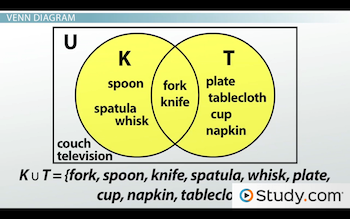Highlighting sets in a Venn diagram shows union.

### Intersection

K intersects T = {fork, knife}.

To show intersection in a Venn diagram, we highlight only where the two circles intersect each other in the middle of our picture: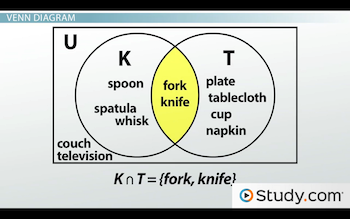Highlighting only the portions of the sets that cross each other in a Venn diagram shows intersection.

### Complement

K' = {plate, cup, napkin, tablecloth, television, couch}.

To show complement in a Venn diagram, we highlight everything except the circle that represents set K: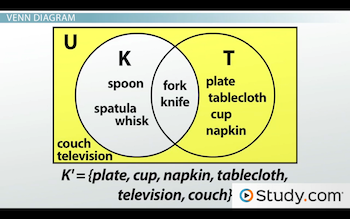Highlighting everything outside the circle you are looking at shows complement.

### Subset

Let's pretend we have another set called B = {spatula, whisk}, elements we would need to bake. That means in our Venn diagram we need another circle inside of K.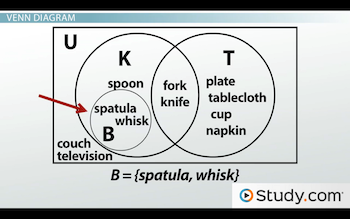A subset shows up as a circle within a circle in a Venn diagram.

Now, we can say B is a subset of K because every element in B is also in set K. Using a Venn diagram, we see a circle within a circle.

### Disjoint

Let's look at the elements {couch, television}. How about we call them set P = {couch, television}. We can see that circle P isn't inside of circles K or T. It also doesn't intersect any of those sets:Drawing a set outside of any other sets shows disjoint on a Venn diagram.

P does not intersect K, or, P intersects K = the empty set.

The symbol that looks like a zero with a line through it means the empty set. There isn't any element that is in P and K at the same time, so it's empty!

This set P is called a disjoint set. It is disjoint, or has no elements that are the same in any other set, but it is still in our universal set.

### Lesson Summary

K union T = {fork, spoon, knife, spatula, whisk, plate, cup, napkin, tablecloth}. Union is an object in set K(the kitchen) or set T (on the table). Set B is also part of set K, so it must be highlighted, too.

K intersects T = {fork, knife}. Intersection is any object that is in the kitchen and on the table at the same time.

K' = {plate, cup, napkin, tablecloth, television, couch}. Complement is any element that is not in the set listed. So, K' is all elements not in set K.

### Next Topics